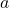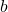One of my favorite topics to teach is quadratic inequalities, which are usually taught in Algebra 2 and/or Precalculus classes. I enjoy presenting a question to my students and then exploring different paths to get to the solution. I usually start the lesson by scaffolding some of the skills the students will need such as factoring and solving linear inequalities. At that point, I ask students a general thinking question “If the product of two numbers a and b is negative, what does that tell you about the numbers?”.

After a brief discussion, students will arrive to the same conclusion:

• “One number is positive and the other is negative”
• “The numbers have different signs”
• “They can’t have the same sign, otherwise the product is positive”

We then rewrite their conclusion using mathematical notation. If the product ofis negative, then there are two possible cases:

1.is negative andis positive2.is positive andis negativeAt this point, we are ready to begin exploring quadratic inequalities. We begin with, students factor and find the x-values that make the inequality equal to zero (in this case). Then using sign analysis on a number line, students will figure out on which interval(s) is the product of the two factors negative. In our example, the interval that satisfies the inequality goes from negative two to positive two. Students can write their solution in interval notation or as an inequality. This is probably the most commonly used method to solve a quadratic inequality among high school students. Pictured below is what the solution looks like for this method:After using the sign analysis on a number line, we then transition to a graphic approach of the problem. Students will graph the functionand will pay attention to the x-intercepts. Students will notice that the x-intercepts are locatedand that the graph of the function is less than zero (or below the x-axis) between negative two and positive two. The graphic solution provides students with a different lens that deepens their understanding.Finally, students will approach and solve the question as a compound inequality. Since the left side of the inequality is already factored, we will consider the two possible cases mentioned at the beginning of the lesson. First, we will consider, when we solve the inequalities the solution is any number greater than or equal to negative two and less than or equal to positive two. In other words, everything between negative two and positive two inclusive. This solution is consistent with our sign analysis and the graphic approach.

When we consider the second case, we end up with an impossible solution. We need a number greater than or equal to two and less than or equal to negative two. Since there is no number that satisfies both conditions, we say there is no solution.After exploring all three methods, I share with students that sign analysis will probably be more convenient to use as we go into problems that involve higher degrees and/or division. Showing different methods to solve a single question takes time and it’s not always feasible. However, whenever possible, I believe that exposing students to multiple approaches gives them a more solid understanding of the mathematics they are learning.